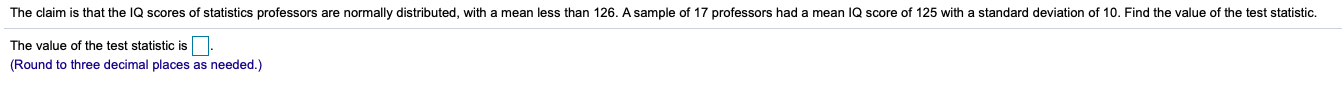that the IQ scores of statisticss professors are normally distributed, with a mean less than 126. A sample of 17 professors had a mean IQ score of 125 with a standard deviation of 10. Find the value of the test statisticThe claimThe value of the test statistic is(Round to three decimal places as needed.)

Question

The claim is that the IQ scores of statistics professors are normally​ distributed, with a mean less than 126.A sample of 17 professors had a mean IQ score of 125 with a standard deviation of 10.Find the value of the test statistic.help_outlineImage Transcriptionclosethat the IQ scores of statisticss professors are normally distributed, with a mean less than 126. A sample of 17 professors had a mean IQ score of 125 with a standard deviation of 10. Find the value of the test statistic The claim The value of the test statistic is (Round to three decimal places as needed.) fullscreen
Step 1

In this question, A researcher claims that IQ scores of Statistics professors is less than 126, for the study researcher collects a samp...

Want to see the full answer?

See Solution

Want to see this answer and more?

Our solutions are written by experts, many with advanced degrees, and available 24/7

See Solution
Tagged in

Hypothesis Testing